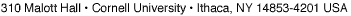## MATH 6620: Riemannian Geometry (Spring 2010)

Instructor: Xiaodong Cao

This course will be an introduction to Riemannian geometry. We will cover the following topics: linear connections, Riemannian metric and parallel translation, covariant derivative and curvature tensors, the exponential map, the Gauss lemma and completeness of the metric, isometries and space forms, Jacobi fields and the theorem of Cartan-Hadamard, the first and second variation formulas, the index form of Morse and the theorem of Bonnet-Myers, the Rauch, Hessian, and Laplacian comparison theorems, the Morse index theorem, the conjugate and cut loci, submanifolds and the second fundamental form.